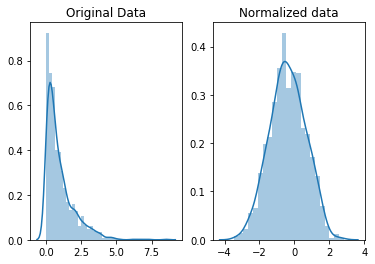## ROC Curve and AUCROC curves and AUC are used to measure performance in machine earning. They are the most widely used evaluation metrics for checking any classification model’s performance. It tells how much the model is capable of distinguishing between classes. ROC (Receiver Operator Characteristic Curve) is a probability curve and AUC represents the…

## Logistic RegressionLogistic Regression is a supervised classification algorithm that is used to predict the probability of a categorical dependent variable using a given set of independent variables. It is a predictive analysis algorithm and based on the concept of probability. The most common use of logistic regression models is in binary classification problems. Some…

## Gauss Markov Theorem: OLS is BLUE!

The Gauss-Markov theorem states that if your linear regression model satisfies the classical assumptions, then ordinary least squares (OLS) regression produces best linear unbiased estimates (BLUE) that have the smallest variance of all possible linear estimators. There are five Gauss Markov assumptions: Linearity: the parameters we are estimating using the OLS method must be themselves linear. Random:…

## Scaling- Normalization vs StandardizationFeature scaling is an important technique in Machine Learning and it is one of the most important steps during the preprocessing of data before creating a machine learning model. The reason to perform features scaling is to ensure one feature doesn’t dominate others. The two most important scaling techniques are…

## Coefficient of Correlation vs Coefficient of Determination

Coefficient of Correlation: It is the degree of relationship between two variables. Any two variables in this universe can be argued to have a correlation value. If they are not correlated then the correlation value can still be computed which would be 0. The correlation value always lies between -1…

## Reinforcement Learning

The reason why I included reinforcement learning in this article, is that one might think that “supervised” and “unsupervised” encompass every ML algorithm, and it actually does not. There are algorithms that aren’t supervised nor unsupervised, like Reinforcement Learning. Reinforcement learning is the field that studies the problems and techniques…

## ML Algorithms

I will discuss the most popular machine learning algorithms. There are so many algorithms available and I categorized algorithms based on the learning style. Let’s take a look at four different learning styles in machine learning algorithms: Supervised Learning Unsupervised Learning Semi-supervised Learning Reinforcement Learning Here is the list of…

## Supervised Learning

Supervised learning is a class of ML where machines can learn through examples. The historical data set has both input and output (also called target or response) values. Based on the training data set, the machine already has some idea of what the target value would look like, which kind…